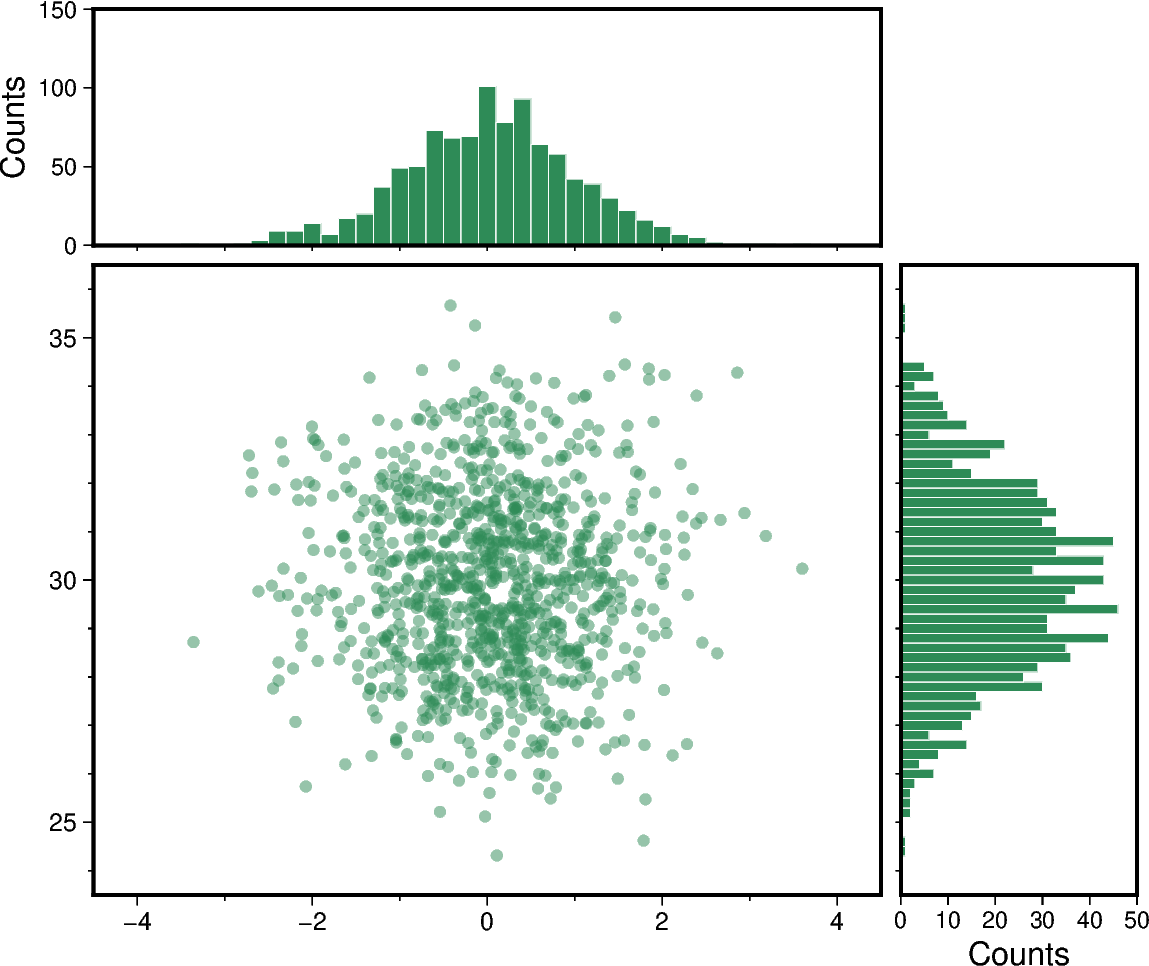# Scatter plot with histograms

To create a scatter plot with histograms at the sides of the plot one can use `pygmt.Figure.plot` in combination with `pygmt.Figure.histogram`. The positions of the histograms are plotted by offseting them from the main scatter plot figure using `pygmt.Figure.shift_origin`.```import numpy as np
import pygmt

np.random.seed(19680801)

# Generate random data from a standard normal distribution centered on 0
x = np.random.randn(1000)
y = np.random.randn(1000)

# Get axis limits
xymax = max(np.max(np.abs(x)), np.max(np.abs(y)))

fig = pygmt.Figure()
fig.basemap(
region=[-xymax - 0.5, xymax + 0.5, -xymax - 0.5, xymax + 0.5],
projection="X10c/10c",
frame=["WSrt", "a1"],
)

fillcol = "seagreen"

# Plot data points as circles with a diameter of 0.15 centimeters
fig.plot(x=x, y=y, style="c0.15c", fill=fillcol, transparency=50)

# Shift the plot origin and add top margin histogram
fig.shift_origin(yshift="10.25c")

fig.histogram(
projection="X10c/2c",
frame=["Wsrt", "xf1", "y+lCounts"],
# Give the same value for ymin and ymax to have ymin and ymax
# calculated automatically
region=[-xymax - 0.5, xymax + 0.5, 0, 0],
data=x,
fill=fillcol,
pen="0.1p,white",
histtype=0,
series=0.1,
)

# Shift the plot origin and add right margin histogram
fig.shift_origin(yshift="-10.25c", xshift="10.25c")

fig.histogram(
horizontal=True,
projection="X2c/10c",
# Note that the y-axis annotation "Counts" is shown in x-axis direction
# due to the rotation caused by horizontal=True
frame=["wSrt", "xf1", "y+lCounts"],
region=[-xymax - 0.5, xymax + 0.5, 0, 0],
data=y,
fill=fillcol,
pen="0.1p,white",
histtype=0,
series=0.1,
)

fig.show()
```

Total running time of the script: ( 0 minutes 1.120 seconds)

Gallery generated by Sphinx-Gallery